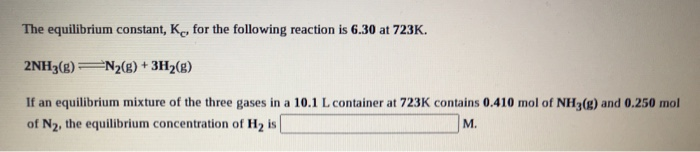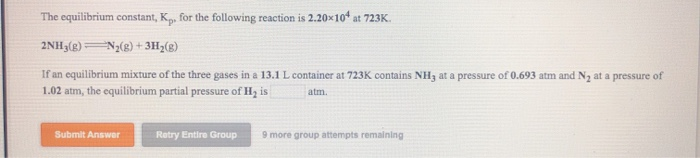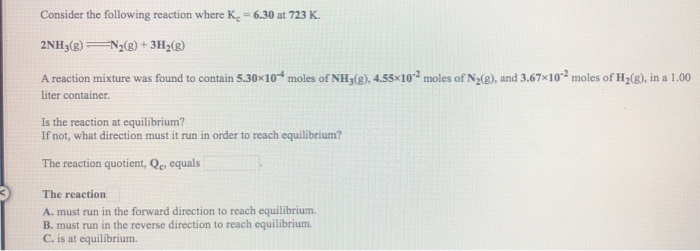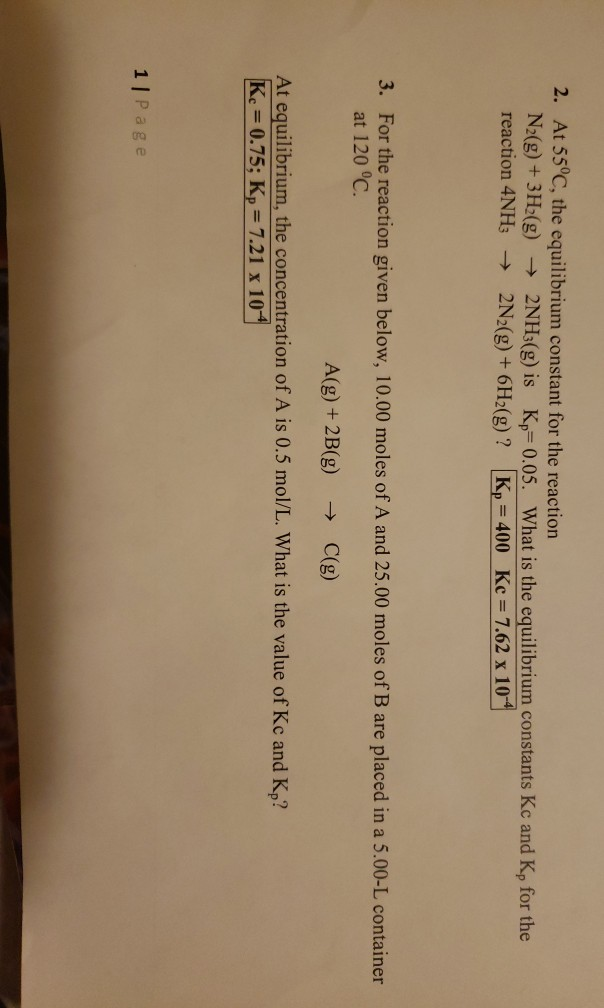Question

# The equilibrium constant, Kc, for the following reaction is 6.30 at 723K. 2NH3(g) N2(g) + 3H2(g)...

The equilibrium constant, Kc, for the following reaction is 6.30 at 723K. 2NH3(g) N2(g) + 3H2(g) If an equilibrium mixture of the three gases in a 15.7 L container at 723K contains 0.284 mol of NH3(g) and 0.437 mol of N2, the equilibrium concentration of H2 is__________ M.

[NH3] = mol of NH3 / volume in L

= 0.284 mol / 15.7 L

= 0.01809 M

[N2] = mol of N2 / volume in L

= 0.437 mol / 15.7 L

= 0.02783 M

Now use:

Kc = [N2][H2]^3 / [NH3]^2

6.30 = (0.02783) * [H2]^3 / (0.01809)^2

[H2]^3 = 0.07408

[H2] = 0.420 M

#### Earn Coins

Coins can be redeemed for fabulous gifts.

Similar Homework Help Questions
• ### The equilibrium constant, K, for the following reaction is 6.30 at 723K. 2NH3(g) N2(g) + 3H2(g)...The equilibrium constant, K, for the following reaction is 6.30 at 723K. 2NH3(g) N2(g) + 3H2(g) If an equilibrium mixture of the three gases in a 10.1 L container at 723K contains 0.410 mol of NH3(g) and 0.250 mol of N2, the equilibrium concentration of Hy is M.

• ### The Kc for the following reaction at 225 ∘C is 1.7×102. 3H2(g)+N2(g)⇌2NH3(g) If the equilibrium mixture...

The Kc for the following reaction at 225 ∘C is 1.7×102. 3H2(g)+N2(g)⇌2NH3(g) If the equilibrium mixture contains 0.19 M H2 and 0.021 M N2, what is the molar concentration of NH3?

• ### The equilibrium constant, K. for the following reaction is 2.20x104 at 723K 2NH3(8) N2(g) + 3H2(8)...The equilibrium constant, K. for the following reaction is 2.20x104 at 723K 2NH3(8) N2(g) + 3H2(8) If an equilibrium mixture of the three gases in a 13.1 L container at 723K contains NH, at a pressure of 0.693 atm and Ny at a pressure of 1.02 atm, the equilibrium partial pressure of His atm. Submit Answer Retry Entire Group 9 more group attempts remaining

• ### For the reaction 3H2(g)+N2(g)⇌2NH3(g) at 225 ∘C the equilibrium contant is 1.7×102. If the equilibrium mixture...

For the reaction 3H2(g)+N2(g)⇌2NH3(g) at 225 ∘C the equilibrium contant is 1.7×102. If the equilibrium mixture contains 0.24 M H2 and 0.017 M N2, what is the molar concentration of NH3?

• ### For the reaction N2(g)+3H2(g)⇌2NH3(g) what is the value of Kc at 500 ∘C if the equilibrium...

For the reaction N2(g)+3H2(g)⇌2NH3(g) what is the value of Kc at 500 ∘C if the equilibrium concentrations are as follows: [H2] = 0.45 M , [N2] = 0.50 M , and [NH3] = 2.0 M ?

• ### For the reaction N2(g)+3H2(g)⇌2NH3(g) what is the value of Kc at 500 ∘C if the equilibrium...

For the reaction N2(g)+3H2(g)⇌2NH3(g) what is the value of Kc at 500 ∘C if the equilibrium concentrations are as follows: [H2] = 0.35 M , [N2] = 0.40 M , and [NH3] = 1.8 M ?how to solve answer?

• ### Consider the chemical reaction 2NH3(g) ó N2(g) + 3H2(g). The equilibrium is to be established in...

Consider the chemical reaction 2NH3(g) ó N2(g) + 3H2(g). The equilibrium is to be established in a 50.0 L container at 1,000 K, where Kc = 4.0 × 10-2. Initially, 6.10 x 105 moles of NH3(g) are present. Calculate the amount of H2 presentat equilibrium. [H2] =_____

• ### Calculate KC in terms of molar concentration for the reaction N2(g) + 3H2(g)   2NH3(g) when the...

Calculate KC in terms of molar concentration for the reaction N2(g) + 3H2(g)   2NH3(g) when the equilibrium concentration moles per liter are: N2 = 0.02, H2 = 0.01, NH3 = 0.10.

• ### Consider the following reaction where K. = 6.30 at 723 K. 2NH3(B) = N2(g) + 3H2() A reaction mixture was found to c...Consider the following reaction where K. = 6.30 at 723 K. 2NH3(B) = N2(g) + 3H2() A reaction mixture was found to contain 5.30*10* moles of NH3(g), 4.55*10moles of N2(8), and 3.67x10-2 moles of H2(e), in a 1.00 liter container Is the reaction at equilibrium? If not, what direction must it run in order to reach equilibrium? The reaction quotient, Qc, equals The reaction A. must run in the forward direction to reach equilibrium B. must run in the reverse...

• ### 2. At 55°C, the equilibrium constant for the reaction N2(g) + 3H2(g) + 2NH3(g) is Kp=0.05....2. At 55°C, the equilibrium constant for the reaction N2(g) + 3H2(g) + 2NH3(g) is Kp=0.05. What is the equilibrium constants Kc and Kp for the reaction 4NH3 + 2N2(g) + 6H2(g)? Kp = 400 Kc = 7.62 x 10 3. For the reaction given below, 10.00 moles of A and 25.00 moles of B are placed in a 5.00-L container at 120 °C. A(g) +2B(g) → C(g) At equilibrium, the concentration of A is 0.5 mol/L. What is the...